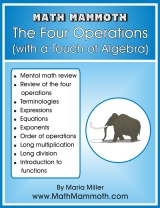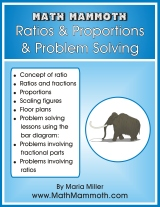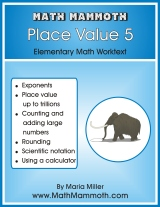### New Math Mammoth books

There are now three new books available in the Blue Series. They are all for grades 5-6. The material for these books came from the Light Blue 5th and 6th grade curricula. Please click on the links to learn more and see samples.

1.### Math Mammoth The Four Operations(with a Touch of Algebra)

The main topics studied in this book are simple equations, expressions that involve a variable, the order of operations, long multiplication, long division, and graphing simple linear functions.

The idea is not to practice each of the four operations separately, but rather to see how they are used together in solving problems and in simple equations. We are trying to develop student's algebraic thinking. Many of the ideas in this chapter are preparing them for algebra in advance.

2. ### Math Mammoth Ratios & Proportions & Problem SolvingThis worktext concentrates, first of all, on two important concepts: ratios and proportions, and then on problem solving.

First, we study thoroughly the concept of ratio, including how it connects with fractions and the aspect ratio in rectangles.

Solving proportions is divided into three separate lessons. In the first one, we solve proportions by thinking through equivalent ratios. In the second one, the usual method of cross-multiplying is introduced. Then follows a lesson that explains just why cross-multiplying is allowed. Then there is more practice with solving proportions and word problems. We also study scaling geometric figures and floor plans.

The last lessons concentrate on various kinds of word problems that can be solved with the help of bar or block diagrams (the same kind as in Singapore Math). These involve problems with fractional parts, and then problems involving ratios. The diagrams become a powerful tool to solve such problems without the use of algebra.

3.### Math Mammoth Place Value 5

Math Mammoth Place Value 5 is a short worktext that covers value up to the trillions—that is, numbers up to 15 digits—rounding them, and using a calculator.

The first lesson covers place value up to billions. Then, we study exponents, and right after that, place value up to trillions, writing the numbers in the expanded form using exponents. After working with addition a little, there are two lessons about calculator usage.